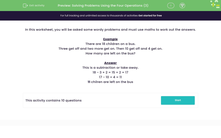# Solving Problems Using the Four Operations (3)

In this worksheet, students use their knowledge of addition, subtraction, multiplication and division to answer number problems.Key stage:  KS 2

Curriculum topic:   Number: Multiplication and Division

Curriculum subtopic:   Solve Multiplication/Division Problems

Difficulty level:#### Worksheet Overview

In this activity, we will work on word problems using addition, subtraction, multiplication, and division.

Before starting to solve a word problem, we must check which operation is needed.

Let's take a look at a word problem together.

Jake has 17 stickers.

Jane has twice as many as Jake.

How many does Jane have?

This question is a multiplication word problem.

We need to double the number of stickers Jake has, to find out how many Jane has.

To double a number, we multiply it by 2.

17 × 2 = 34

Jane has 34 stickers.

Now let's try an example question together.

Example

There are 18 children on a bus.

Three get off and two more get on. Then 10 get off and 4 get on.

How many are left on the bus?

This is a subtraction problem.

18 - 3 + 2 = 15 + 2 = 17

17 - 10 + 4 = 11

11 children are left on the bus.

Now you're ready to try some questions by yourself.

### What is EdPlace?

We're your National Curriculum aligned online education content provider helping each child succeed in English, maths and science from year 1 to GCSE. With an EdPlace account you’ll be able to track and measure progress, helping each child achieve their best. We build confidence and attainment by personalising each child’s learning at a level that suits them.

Get started